## Newton Raphson using Microsoft Excel

Newton Raphson method is one of the most famous numerical methods to find root of equation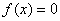. The algorithm is iterative using difference equation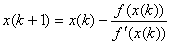You need to find initial value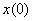near to the solution.

To demonstrate the method, we will use MS Excel in two ways to do the iteration.

The first way is using spreadsheet and the second way is using MS Excel iteration.

Example, we want to find the square root of a number, say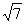. Let us name this number as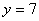. Let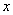be the square root we are looking for, then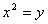or equivalent to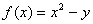. The first derivative is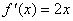. To solve this using spreadsheet table, we make a table as shown in the figure below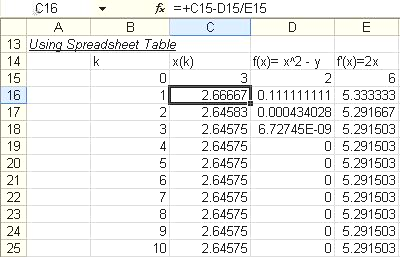We set any initial value say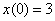and compute theand. Then, we use Equation 1 to calculate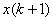. Copy and paste these formulas to many rows below, then you get the result becauseis tend to be convergence to a number of 2.6457.

To use MS Excel iteration, first, use Tools-Options: Calculation Tab, check Iteration check box

1. Type the formulas of,and input x(k) = x(0) as the initial value. The result is shown in the figure below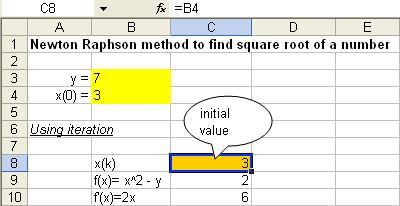2. After that, type the formula of Newton Raphson in C8 = C8-C9/C10

3. Press F9 to recalculate. You get the result as shown in the figure below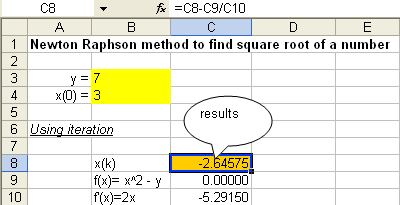Note that most of iteration procedures are sensitive to initial condition. For example, if you input the initial condition of -5, you may get negative result as the square root which is incorrect.

Unlike spreadsheet way, the iteration procedure is only work for one time usage only. Next time you want to recalculate, you need to do all the steps above again.

Preferable reference for this tutorial is

Teknomo, Kardi (2015) Newton Raphson Using Microsoft Excel. https:\\people.revoledu.com\kardi\ tutorial\Excel\NewtonRaphson.html